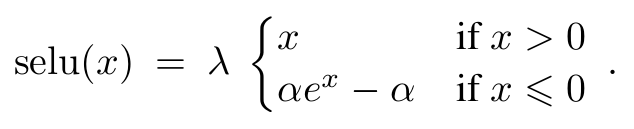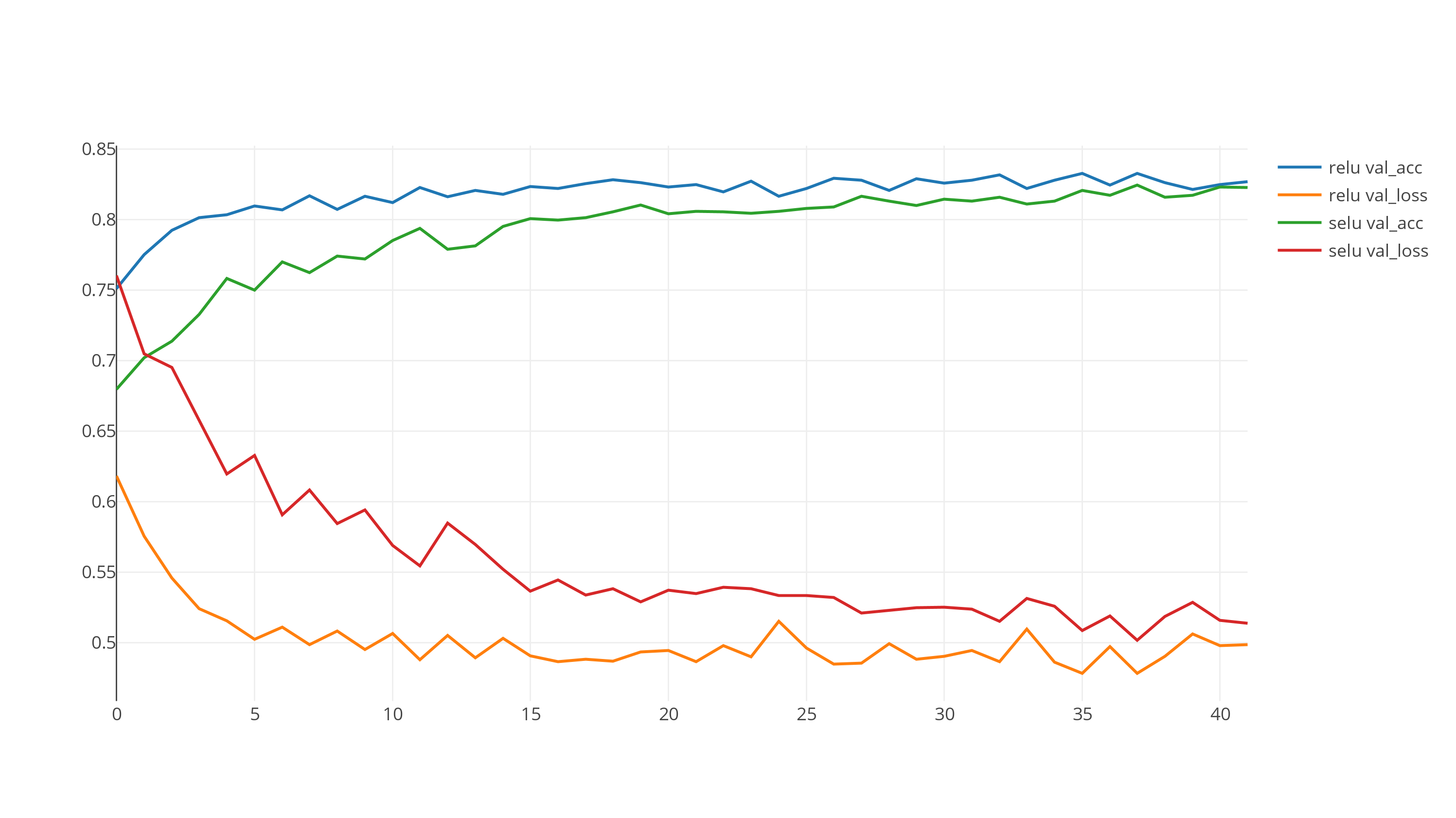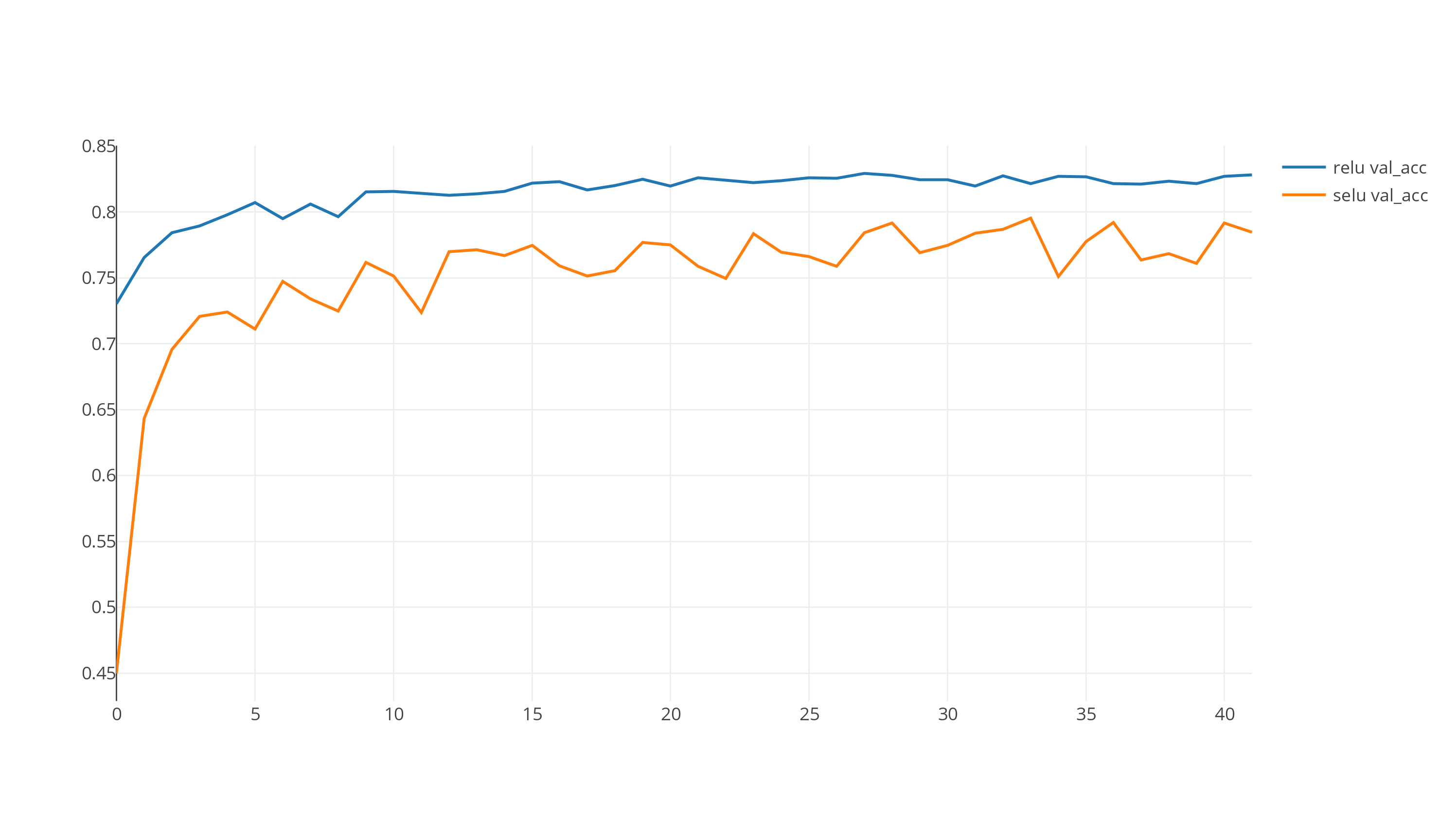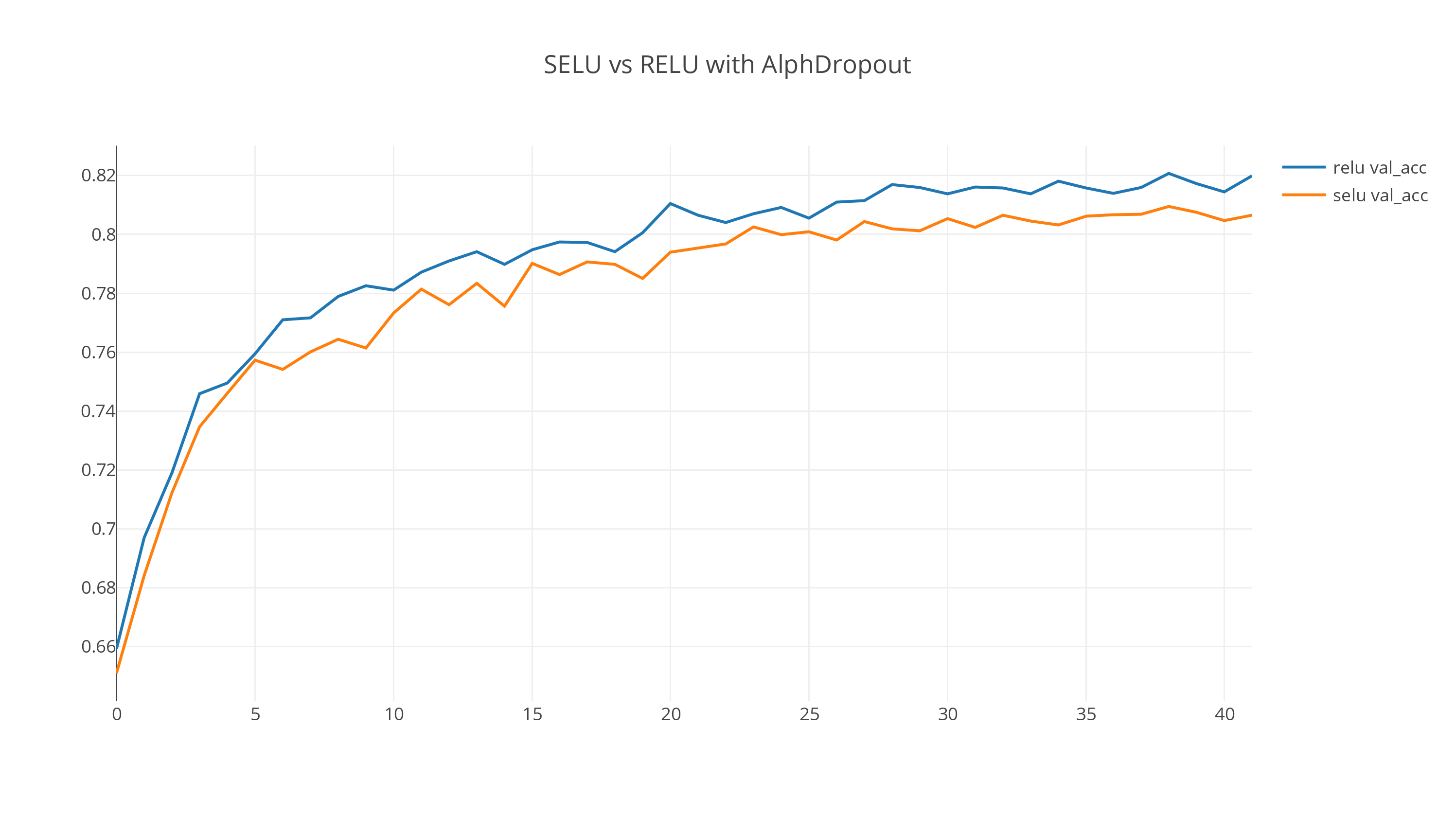# SELU vs RELU activation in simple NLP models

### Background on SELU

Normalized outputs seem to be really helpful in stabilizing the training process. That’s the main reason behind the popularity of BatchNormalization. SELU is a way to output the normalized activations to the next layer.

The overall function is really simple:For `mean 0` and `stdev 1` inputs, the values of α and λ come out to be `1.6732632423543772848170429916717` and `1.0507009873554804934193349852946` respectively.

``````# PyTorch implementation
import torch.nn.functional as F

def selu(x):
alpha = 1.6732632423543772848170429916717
scale = 1.0507009873554804934193349852946
return scale * F.elu(x, alpha)
``````
``````# Numpy implementation
import numpy as np

def selu(x):
alpha = 1.6732632423543772848170429916717
scale = 1.0507009873554804934193349852946
return scale * ((x > 0)*x + (x <= 0) * (alpha * np.exp(x) - alpha))
``````

### SNLI dataset

SNLI dataset is a collection of 570k english sentence pairs. The task is to classify each pair as either:

• entailment - “A soccer game with multiple males playing.” and “Some men are playing a sport.”
• contradiction - “A man inspects the uniform of a figure in some East Asian country.” and “The man is sleeping.”
• neutral - “A smiling costumed woman is holding an umbrella.” and “A happy woman in a fairy costume holds an umbrella.”

### Model Architecture

I am using a simple Bag of words model written in keras. The following python snippet describes the major components. This code is taken from Stephen Merity’s repo here.

``````# Embedding layer
embed = Embedding(VOCAB, EMBED_HIDDEN_SIZE, weights=[embedding_matrix], input_length=MAX_LEN, trainable=False)
# A dense layer applied over each sequence point
translate = TimeDistributed(Dense(SENT_HIDDEN_SIZE, activation=ACTIVATION))
# A layer to sum up the sequence of words
rnn = keras.layers.core.Lambda(lambda x: K.sum(x, axis=1), output_shape=(SENT_HIDDEN_SIZE, ))

# 2 pairs of input sentences
premise = Input(shape=(MAX_LEN, ), dtype='int32')
hypothesis = Input(shape=(MAX_LEN, ), dtype='int32')
# Get the word embeddings for each of these 2 pairs
prem = embed(premise)
hypo = embed(hypothesis)
# Apply the Dense layer
prem = translate(prem)
hypo = translate(hypo)
# Sum up the sequence
prem = rnn(prem)
hypo = rnn(hypo)
prem = BatchNormalization()(prem)
hypo = BatchNormalization()(hypo)
# Combined the 2 sentences
joint = concatenate([prem, hypo])
joint = Dropout(DP)(joint)
# Add Few dense layers in the end
for i in range(3):
joint = Dense(2 * SENT_HIDDEN_SIZE, activation=ACTIVATION, kernel_regularizer=l2(L2)(joint)
joint = Dropout(DP)(joint)
joint = BatchNormalization()(joint)
``````

### SELU vs RELU results

Code for this excercise is available in this repo.`RELU` is clearly converging much faster than `SELU`. My first was to remove the `BatchNormalization` and do the same comparison. The following graph shows the comparison after removing the BatchNorm components.Still, `RELU` seems to be doing a much better job than `SELU` for the default configuration.

This behavior remains more or less the same after iterating through hyperparameters. The following graph is for one of the hyperparameter configurations.(Edit: I incorporated the suggestion from Dan Ofer below and included the graph with `AlphaDropout`.)

To be fair, it is still possible that `SELU` is better in some configurations. Some of the possible reasons are listed below. However, it is clear to me that simply replacing `RELU` with `SELU` isn’t going to improve your existing models.

• `SELU` authors recommend a specific initialization scheme for it to be effective.

Additionally, `SELU` is a bit more computationally expensive than `RELU`. On a g2.2xlarge EC2 instance, `RELU` model took about 49 seconds to complete an epoch, while `SELU` model took 65 seconds to do the same (33% more).